http://en.wikipedia.org/wiki/Huygens%E2%80%93Fresnel_principlehttp://upload.wikimedia.org/wikipedia/commons/thumb/6/60/Refraction_on_an_aperture_-_Huygens-Fresnel_principle.svg/2000px-Refraction_on_an_aperture_-_Huygens-Fresnel_principle.svg.png

Huygens proposed that every point to which a luminous disturbance reaches becomes a source of a spherical wave, and the sum of these secondary waves determines the form of the wave at any subsequent time.Same Huygens-Fresnel principle Refraction on an aperture (slit) but notice the secondary source space equally apart and start from center ending 1/2 space from the edge of slit which is more logical than end at the edge of the slit.Same Animation Huygens-Fresnel principle Refraction on an aperture (slit)

Huygens assumed that the secondary waves traveled only in the "forward" direction and it is not explained in the theory why this is the case. He was able to provide a qualitative explanation of linear and spherical wave propagation, and to derive the laws of reflection and refraction using this principle.

http://electron9.phys.utk.edu/phys136d/modules/m9/diff.htm

Diffraction is a phenomenon whereby waves appear to bend around obstacles, or appear to spread out after passing through a small orifice. The occurrence of
diffraction allows us, for example, to hear sounds from sources that are
hidden from us by some obstacle or other.http://www.physchem.co.za/OB12-wav/diffraction.htm the map reminds me that to easily remember this diffraction occurs when w slit width is comparable to  ? wavelength.

Diffraction is a wave phenomenon and is also observed with water waves in a ripple tank. When wave passes through a small opening, comparable in size to the wavelength, in an obstacle (in this case yellow rectangles) , the wavefront on the other side of the opening resembles the wavefront shown below.http://electron9.phys.utk.edu/phys136d/modules/m9/diff.htm

In the Ejs simulation case,when slit width is comparable in size to the wavelength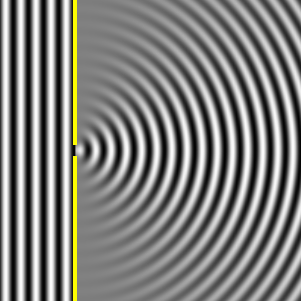same animation when slit width is comparable in size to the wavelength

The wave spreads around the edges of the obstacle (yellow in this case).  This is the phenomenon of diffraction.  Therefore, diffraction is "spreading out " wave phenomenon.

http://electron9.phys.utk.edu/phys136d/modules/m9/diff.htm has some photo of the real ripple tank.http://electron9.phys.utk.edu/phys136d/modules/m9/diff.htm when slit width is comparable in size to the wavelength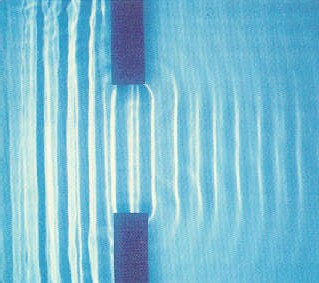http://electron9.phys.utk.edu/phys136d/modules/m9/diff.htm when slit width >> in size than the wavelength

in Ejs,I managed to capture a similar computational model.when slit width is comparable in size to the wavelength assuming 6 secondary sources, diffraction is noticeableslit width >> in size ( w = 6*? ) than the wavelength assuming 10 secondary sources. A wave goes largely straight when the size          of the slit is much larger than the wavelength

I love Phet research and simulation

this is a great simulation called Wave Interference, under the water tab, the simulation breaks the 2D with a side view.

I managed to figure out a cool way to generate a 3D visualization is Ejs.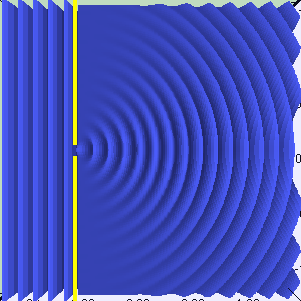when slit width is comparable in size to the wavelength assuming 6 secondary sources, diffraction is noticeable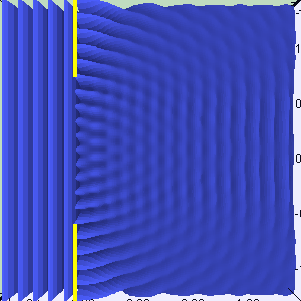slit width >> in size ( w = 6*? ) than the wavelength assuming 10 secondary sources. A wave goes largely straight when the size          of the slit is much larger than the wavelength

my favorable visualization is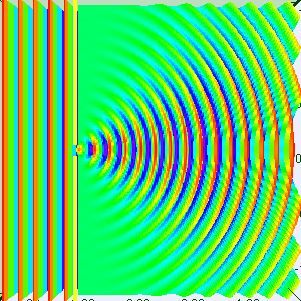Spectrum visualization of when slit width is comparable in size to the wavelength assuming 10 secondary sources, diffraction is noticeable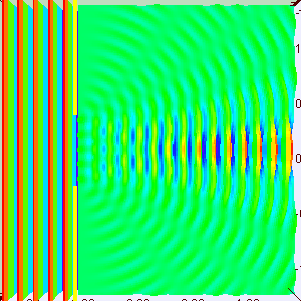Spectrum visualization of slit width >> in size ( w = 5*? ) than the wavelength assuming 10 secondary sources. A wave goes largely straight when the size          of the slit is much larger than the wavelength

other interesting slit simulation are: